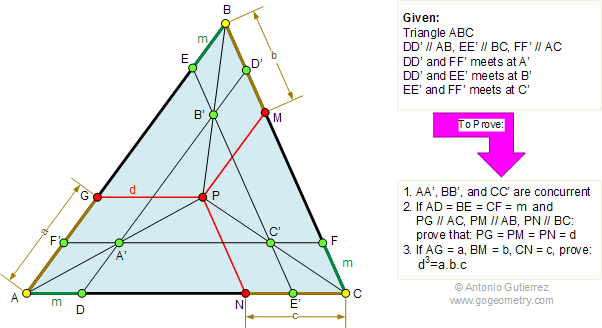Problem 292: Triangle, Parallel, Congruence, Similarity Level: High School, College, SAT Prep. The figure shows a triangle ABC, DD', EE', and FF' are parallel to AB, BC, and AC, respectively. DD', EE', and FF' intersect at A', B', and C', respectively. (1) Prove that AA', BB', and CC' are concurrent at P. (2) If AD = BE = CF = m and PG, PM and PN are parallel to AC, AB, and BC, respectively, prove that PG = PM = PN = d. (3) If AG = a, PM = b, and CN = c, prove that d3 = a.b.c.Recent Additions